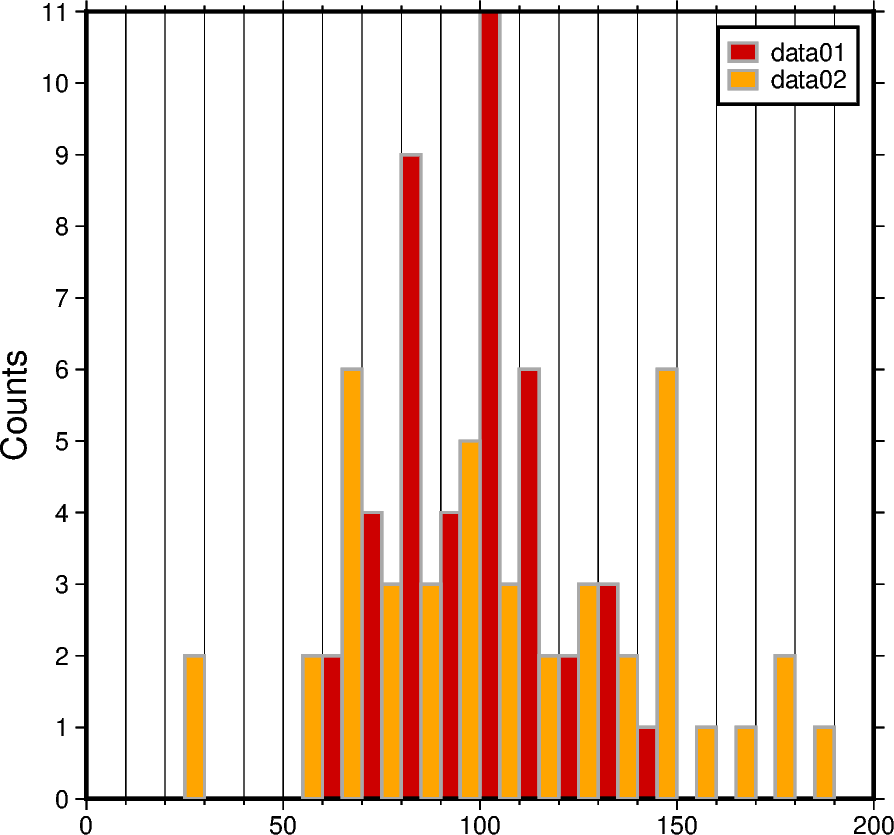# Cartesian histograms

Cartesian histograms can be generated using the `pygmt.Figure.histogram` method. In this tutorial, different histogram related aspects are addressed:

• Using vertical and horizontal bars

• Using stair-steps

• Showing counts and frequency percent

• Adding annotations to the bars

• Showing cumulative values

• Using color and pattern as fill for the bars

• Using overlaid, stacked, and grouped bars

```# Import the required packages
import numpy as np
import pygmt
```

Generate random data from a normal distribution:

```np.random.seed(100)

# Mean of distribution
mean = 100
# Standard deviation of distribution
stddev = 20

# Create two data sets
data01 = np.random.normal(mean, stddev, 42)
data02 = np.random.normal(mean, stddev * 2, 42)
```

## Vertical and horizontal bars

To define the width of the bins, the `series` parameter has to be specified. The bars can be filled via the `fill` parameter with either a color or a pattern (see later in this tutorial). Use the `pen` parameter to adjust width, color, and style of the outlines. By default, a histogram with vertical bars is created. Horizontal bars can be achieved via `horizontal=True`.

```# Create new figure instance
fig = pygmt.Figure()

# Create histogram for data01 with vertical bars
fig.histogram(
# Define the plot range as a list of xmin, xmax, ymin, ymax
# Let ymin and ymax determined automatically by setting both to the same
# value
region=[0, 200, 0, 0],
projection="X10c",  # Cartesian projection with a width of 10 centimeters
# Add frame, annotations (a), ticks (f), and y-axis label (+l) "Counts"
# The numbers give the steps of annotations and ticks
frame=["WStr", "xaf10", "ya1f1+lCounts"],
data=data01,
# Set the bin width via the "series" parameter
series=10,
# Fill the bars with color "red3"
fill="red3",
# Draw a 1-point thick solid outline in "darkgray" around the bars
pen="1p,darkgray,solid",
# Choose counts via the "histtype" parameter
histtype=0,
)

# Shift plot origin 12 centimeters to the right
fig.shift_origin(xshift="12c")

# Create histogram for data01 with horizontal bars
fig.histogram(
region=[0, 200, 0, 0],
projection="X10c",
frame=["WStr", "xaf10", "ya1f1+lCounts"],
data=data01,
series=10,
fill="red3",
pen="1p,darkgray,solid",
histtype=0,
# Use horizontal bars
# Please note the flip of the x and y axes regarding annotations, ticks,
# gridlines, and axis labels
horizontal=True,
)

fig.show()
```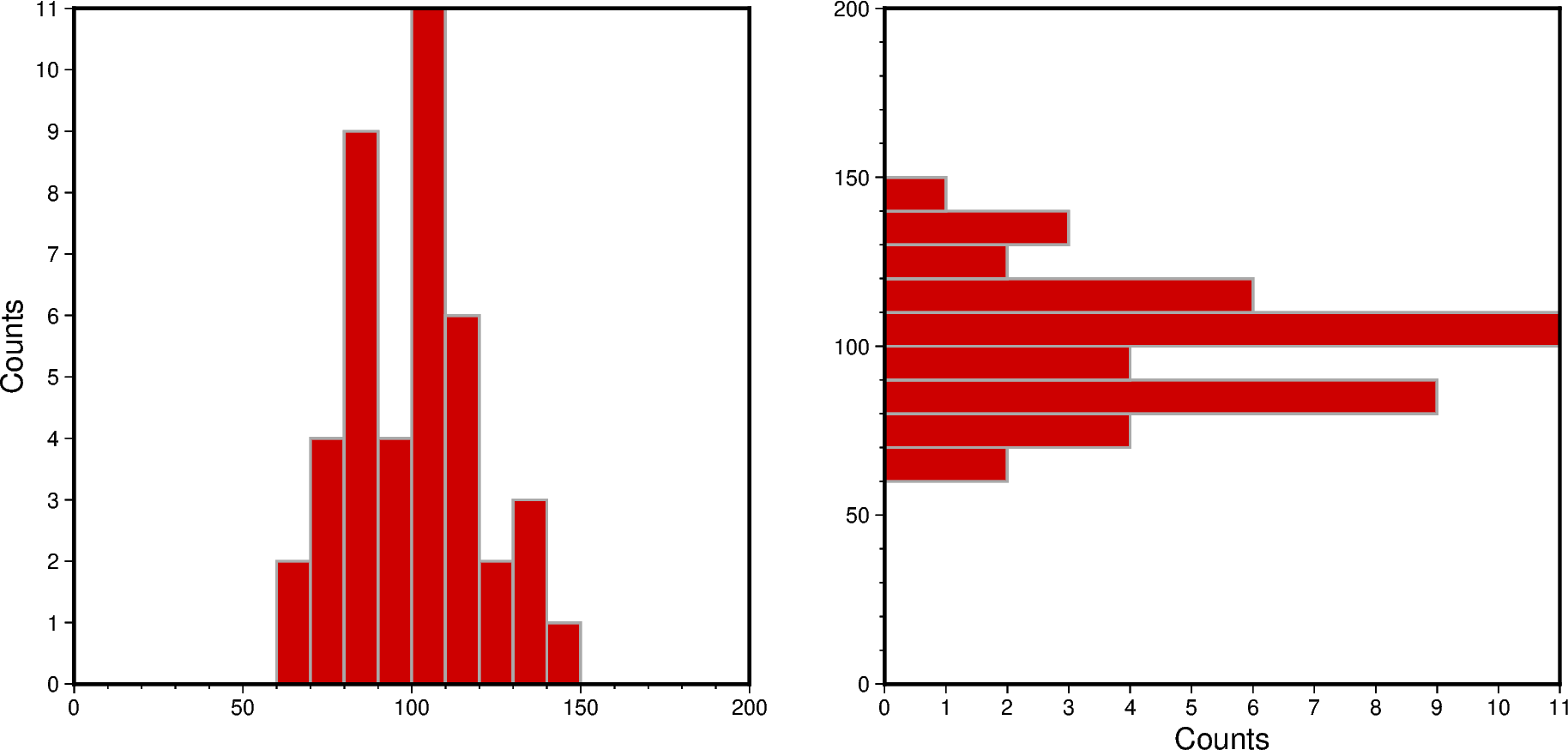## Stair-steps

A stair-step diagram can be created by setting `stairs=True`. Then only the outer outlines of the bars are drawn, and no internal bars are visible.

```# Create new figure instance
fig = pygmt.Figure()

# Create histogram for data01
fig.histogram(
region=[0, 200, 0, 0],
projection="X10c",
frame=["WSne", "xaf10", "ya1f1+lCounts"],
data=data01,
series=10,
# Draw a 1-point thick dotted outline in "red3"
pen="1p,red3,dotted",
histtype=0,
# Draw stair-steps in stead of bars
stairs=True,
)

# Shift plot origin 12 centimeters to the right
fig.shift_origin(xshift="12c")

# Create histogram for data02
fig.histogram(
region=[0, 200, 0, 0],
projection="X10c",
frame=["WSne", "xaf10", "ya1f1+lCounts"],
data=data02,
series=10,
# Draw a 1.5-point thick dashed outline in "orange"
pen="1.5p,orange,dashed",
histtype=0,
stairs=True,
)

fig.show()
```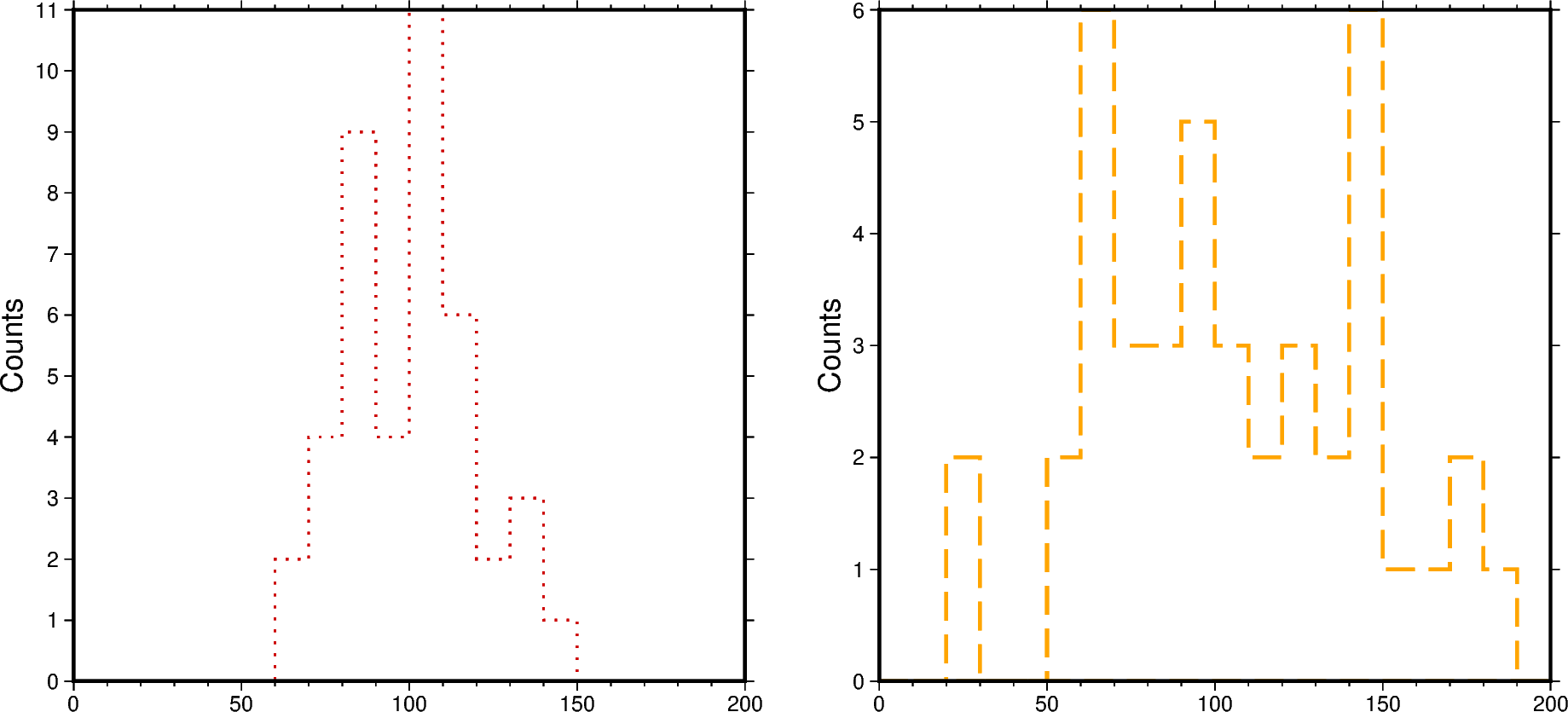## Counts and frequency percent

By default, a histogram showing the counts in each bin is created (`histtype=0`). To show the frequency percent set the `histtpye` parameter to `1`. For further options please have a look at the documentation of `pygmt.Figure.histogram`.

```# Create new figure instance
fig = pygmt.Figure()

# Create histogram for data02 showing counts
fig.histogram(
region=[0, 200, 0, 0],
projection="X10c",
frame=["WSnr", "xaf10", "ya1f1+lCounts"],
data=data02,
series=10,
fill="orange",
pen="1p,darkgray,solid",
# Choose counts via the "histtype" parameter
histtype=0,
)

# Shift plot origin 11 centimeters to the right
fig.shift_origin(xshift="11c")

# Create histogram for data02 showing frequency percent
fig.histogram(
region=[0, 200, 0, 0],
projection="X10c",
frame=["lSnE", "xaf10", "ya2f1+u%+lFrequency percent"],
data=data02,
series=10,
fill="orange",
pen="1p,darkgray,solid",
# Choose frequency percent via the "histtype" parameter
histtype=1,
)

fig.show()
```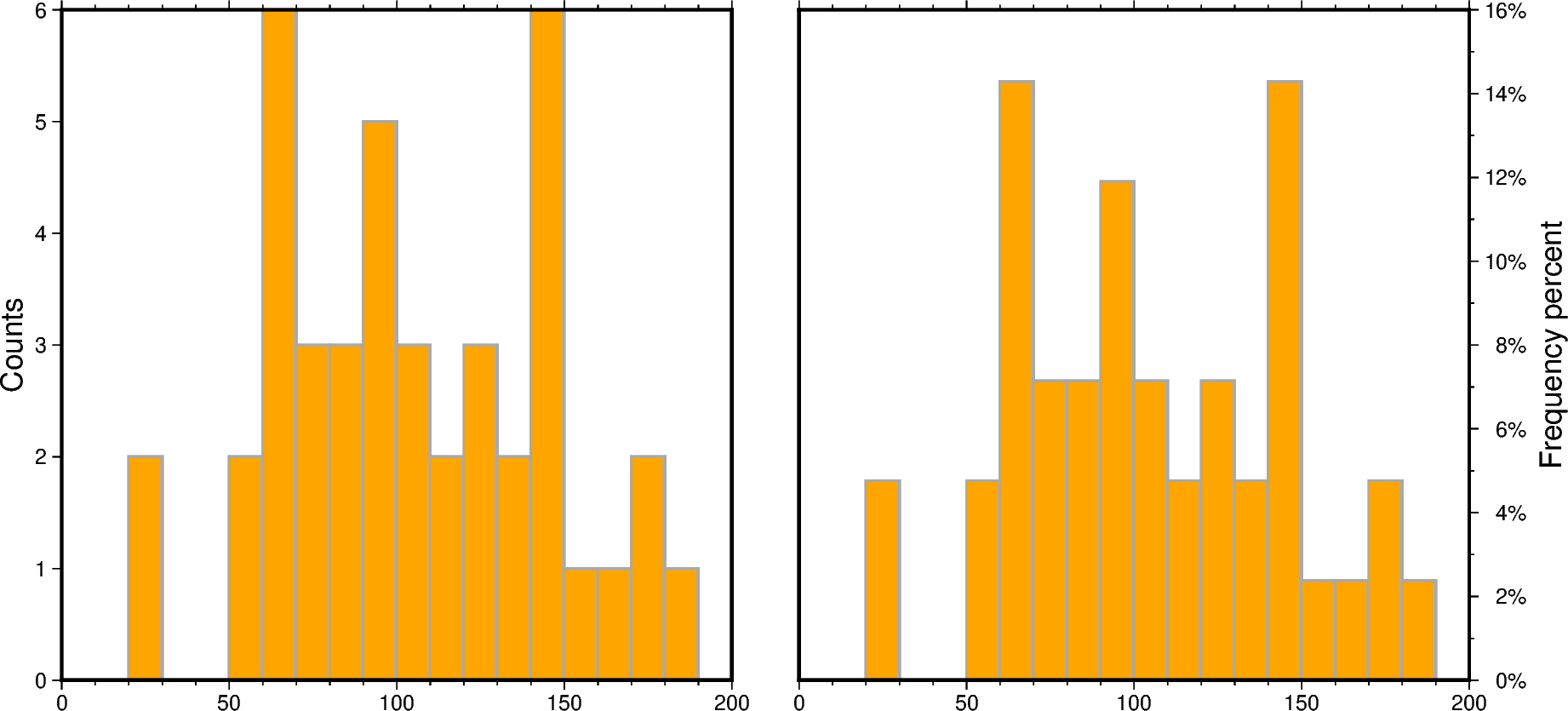## Cumulative values

To create a histogram showing the cumulative values set `cumulative=True`. Here, the bars of the cumulative histogram are filled with a pattern via the `fill` parameter. Annotate each bar with the counts it represents using the `annotate` parameter.

```# Create new figure instance
fig = pygmt.Figure()

# Create histogram for data01 showing the counts per bin
fig.histogram(
region=[0, 200, 0, len(data01) + 1],
projection="X10c",
frame=["WSne", "xaf10", "ya5f1+lCounts"],
data=data01,
series=10,
fill="red3",
pen="1p,darkgray,solid",
histtype=0,
# Annotate each bar with the counts it represents
annotate=True,
)

# Shift plot origin 11 centimeters to the right
fig.shift_origin(xshift="11c")

# Create histogram for data01 showing the cumulative counts
fig.histogram(
region=[0, 200, 0, len(data01) + 1],
projection="X10c",
frame=["wSnE", "xaf10", "ya5f1+lCumulative counts"],
data=data01,
series=10,
# Use pattern (p) number 8 as fill for the bars
# Set the background (+b) to white [Default]
# Set the foreground (+f) to black [Default]
fill="p8+bwhite+fblack",
pen="1p,darkgray,solid",
histtype=0,
# Show cumulative counts
cumulative=True,
# Offset (+o) the label by 10 points in negative y-direction
annotate="+o-10p",
)

fig.show()
```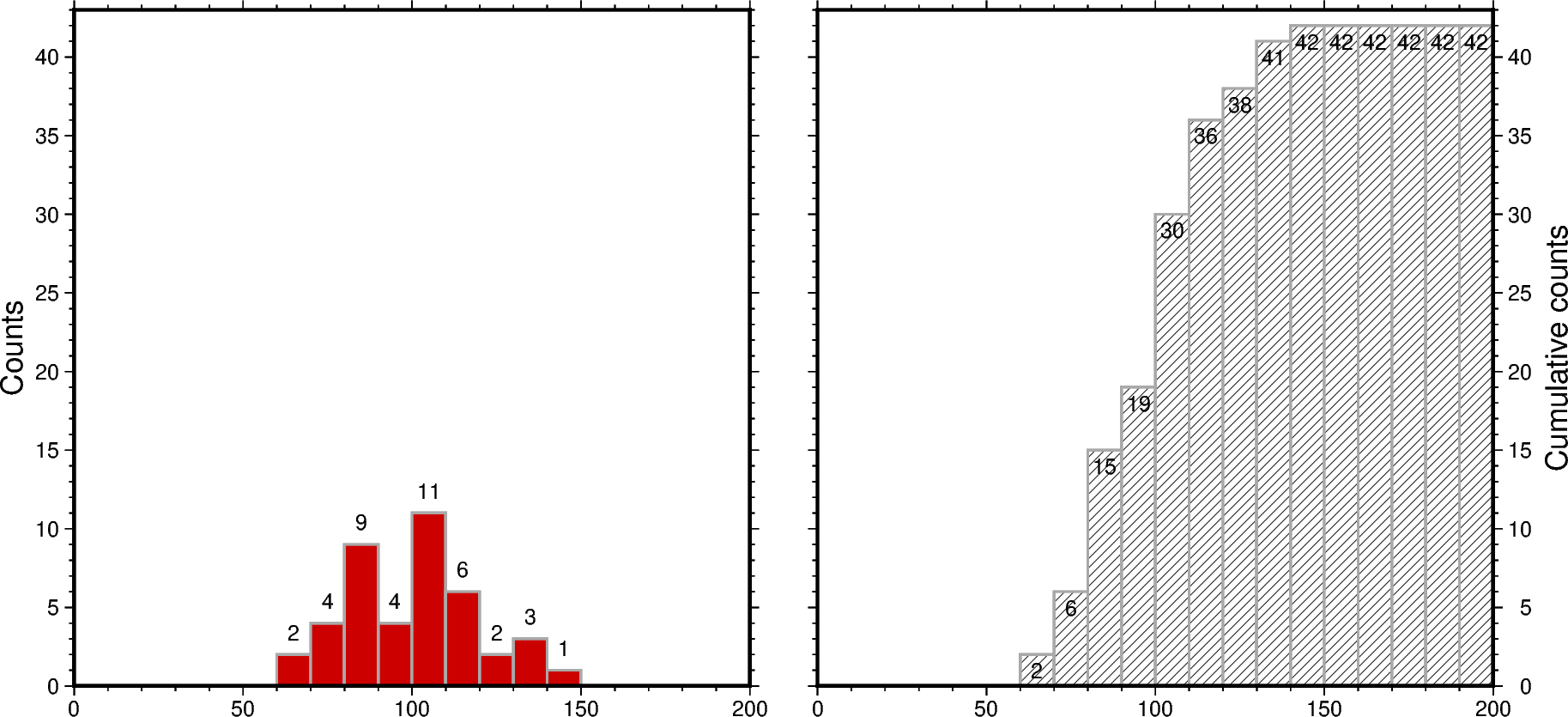## Overlaid bars

Overlaid or overlapping bars can be achieved by plotting two or serveral histograms, each for one data set, on top of each other. The legend entry can be specified via the `label` parameter.

Limitations of histograms with overlaid bars are:

• Mixing of colors or/and patterns

• Visually more colors or/and patterns than data sets

• Visually a “third histogram” (or more in case of more than two data sets)

```# Create new figure instance
fig = pygmt.Figure()

# Create histogram for data01
fig.histogram(
region=[0, 200, 0, 0],
projection="X10c",
frame=["WSne", "xaf10", "ya1f1+lCounts"],
data=data01,
series=10,
fill="red3",
pen="1p,darkgray,solid",
histtype=0,
# Set legend entry
label="data01",
)

# Create histogram for data02
# It is plotted on top of the histogram for data01
fig.histogram(
data=data02,
series=10,
# Fill bars with color "orange", use a transparency of 50% ("@50")
fill="orange@50",
pen="1p,darkgray,solid",
histtype=0,
label="data02",
)

fig.legend()

fig.show()
```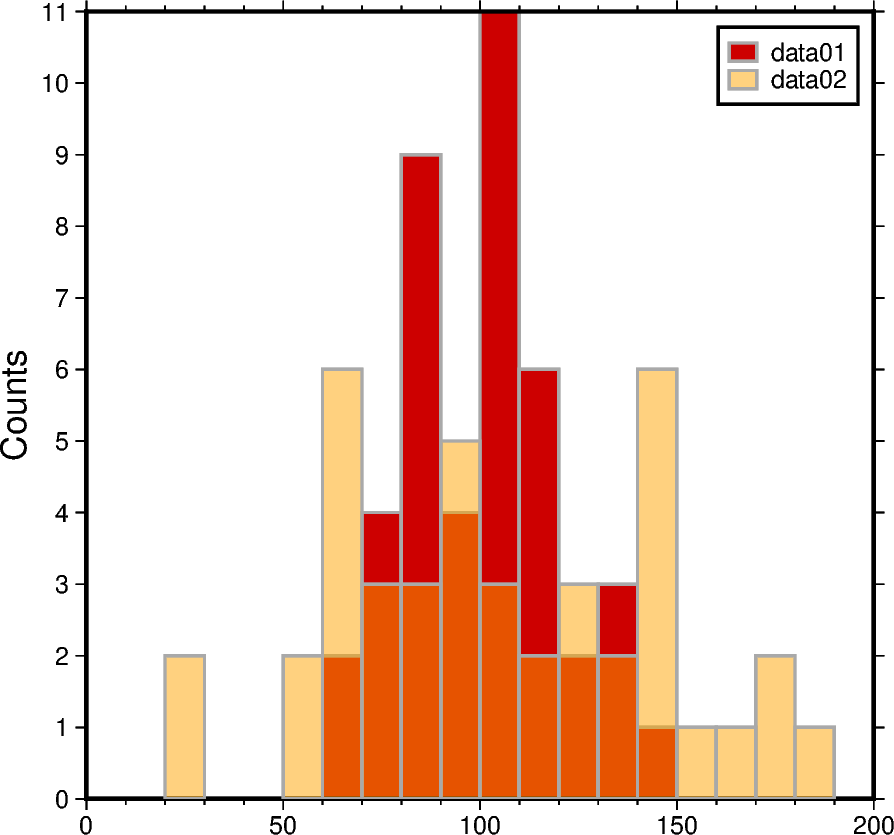## Stacked bars

Histograms with stacked bars are not directly supported by PyGMT. Thus, before plotting, combined data sets have to be created from the single data sets. Then, stacked bars can be achieved similar to overlaid bars via plotting two or several histograms on top of each other.

Limitations of histograms with stacked bars are:

• No common baseline

• Partly not directly clear whether overlaid or stacked bars

```# Combine the two data sets to one data set
data_merge = np.concatenate((data01, data02), axis=None)

# Create new figure instance
fig = pygmt.Figure()

# Create histogram for data02 by using the combined data set
fig.histogram(
region=[0, 200, 0, 0],
projection="X10c",
frame=["WSne", "xaf10", "ya1f1+lCounts"],
data=data_merge,
series=10,
fill="orange",
pen="1p,darkgray,solid",
histtype=0,
# The combined data set appears in the final histogram visually
# as data set data02
label="data02",
)

# Create histogram for data01
# It is plotted on top of the histogram for data02
fig.histogram(
data=data01,
series=10,
fill="red3",
pen="1p,darkgray,solid",
histtype=0,
label="data01",
)

fig.legend()

fig.show()
```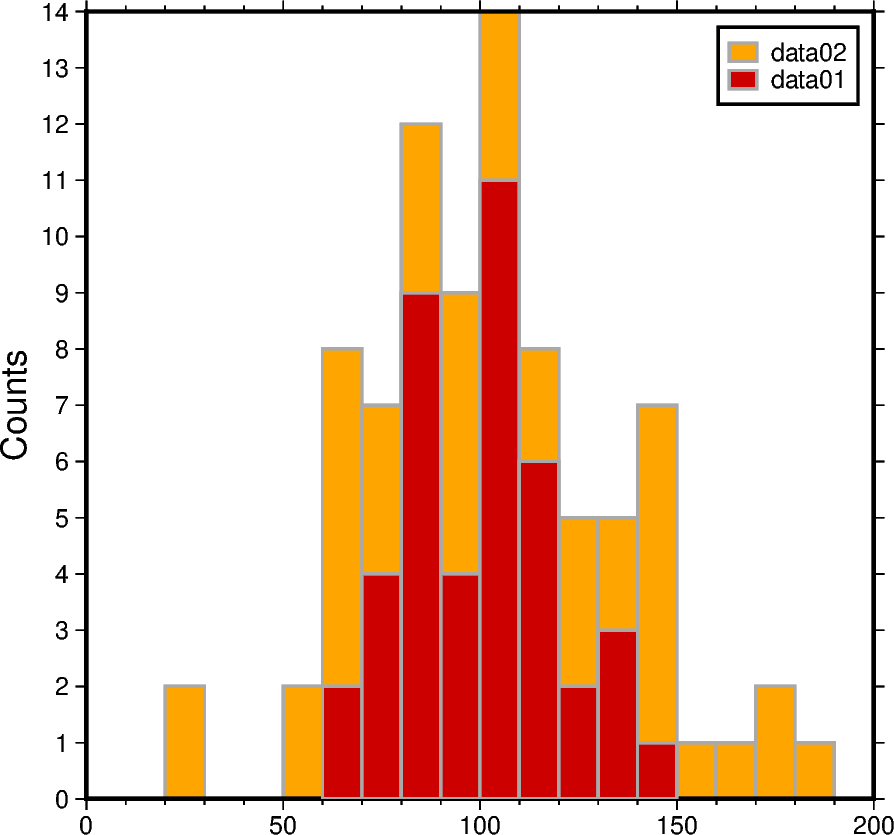## Grouped bars

By setting the `barwidth` parameter in respect to the values passed to the `series` parameter histograms with grouped bars can be created.

Limitations of histograms with grouped bars are:

• Careful setting width and position of the bars in respect to the bin width

• Difficult to see the variations of the single data sets

```# Width used for binning the data
binwidth = 10

# Create new figure instance
fig = pygmt.Figure()

# Create histogram for data01
fig.histogram(
region=[0, 200, 0, 0],
projection="X10c",
frame=["WSne", "xaf10g10", "ya1f1+lCounts"],
data=data01,
series=binwidth,
fill="red3",
pen="1p,darkgray,solid",
histtype=0,
# Calculate the bar width in respect to the bin width, here for two
# data sets half of the bin width
# Offset (+o) the bars to align each bar with the left limit of the
# corresponding bin
barwidth=f"{binwidth/2}+o-{binwidth/4}",
label="data01",
)

# Create histogram for data02
fig.histogram(
data=data02,
series=binwidth,
fill="orange",
pen="1p,darkgray,solid",
histtype=0,
barwidth=f"{binwidth/2}+o{binwidth/4}",
label="data02",
)